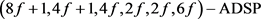# 8阶分圆类构造几乎差集偶Constructions of Almost Difference Set Pairs by Cyclotomic Classes of Order Eight

• 全文下载: PDF(347KB)    PP.172-177   DOI: 10.12677/AAM.2020.92020
• 下载量: 94  浏览量: 150

Almost difference set pairs are widely used in many problems because they are closely related to almost optimal binary sequence pairs. Cyclotomic classes method is an important method to con-struct almost difference set pairs. In this paper, several new almost difference set pairs are con-structed by using cyclotomic classes of order eight.

1. 引言

2. 基础知识

${Z}_{q}^{*}={\cup }_{i=0}^{e-1}{C}_{i}$

3. 8阶分圆类构造几乎差集偶

1) 当f为偶数且2不是4次剩余，且 $b=-y,x-a=4$ 时，

1、 $\left(U,W\right)$ 构成 $\left(8f+1,4f,4f,2f,2f-1,2f\right)-\text{ADSP}$Table 1. Relations of cyclotomic numbers of order 8 for even fTable 2. The fifteen basic cyclotomic numbers of order 8 when f is even and 2 is not a quartic residue

2、 $\left({U}^{\prime },W\right)$ 构成 $\left(8f+1,4f+1,4f,2f,2f,6f\right)-\text{ADSP}$

3、 $\left(U,{W}^{\prime }\right)$ 构成 $\left(8f+1,4f,4f+1,2f,2f,6f\right)-\text{ADSP}$

4、 $\left({U}^{\prime },{W}^{\prime }\right)$ 构成 $\left(8f+1,4f,4f+1,2f+1,2f,2f\right)-\text{ADSP}$

2) 当f为偶数且2不是4次剩余，且 $b=4-y,x-a=4$ 时， $\left(U,W\right)$ 构成 $\left(8f+1,4f,4f,2f,2f-1,2f\right)-\text{ADSP}$

$\begin{array}{c}{\text{Δ}}_{i}=|\left({C}_{0}+{\theta }^{i}\right)\cap {C}_{0}|+|\left({C}_{0}+{\theta }^{i}\right)\cap {C}_{4}|+|\left({C}_{0}+{\theta }^{i}\right)\cap {C}_{5}|+|\left({C}_{0}+{\theta }^{i}\right)\cap {C}_{6}|\\ \text{\hspace{0.17em}}\text{\hspace{0.17em}}+|\left({C}_{1}+{\theta }^{i}\right)\cap {C}_{0}|+|\left({C}_{1}+{\theta }^{i}\right)\cap {C}_{4}|+|\left({C}_{1}+{\theta }^{i}\right)\cap {C}_{5}|+|\left({C}_{1}+{\theta }^{i}\right)\cap {C}_{6}|\\ \text{\hspace{0.17em}}\text{\hspace{0.17em}}+|\left({C}_{2}+{\theta }^{i}\right)\cap {C}_{0}|+|\left({C}_{2}+{\theta }^{i}\right)\cap {C}_{4}|+|\left({C}_{2}+{\theta }^{i}\right)\cap {C}_{5}|+|\left({C}_{2}+{\theta }^{i}\right)\cap {C}_{6}|\\ \text{\hspace{0.17em}}\text{\hspace{0.17em}}+|\left({C}_{4}+{\theta }^{i}\right)\cap {C}_{0}|+|\left({C}_{4}+{\theta }^{i}\right)\cap {C}_{4}|+|\left({C}_{4}+{\theta }^{i}\right)\cap {C}_{5}|+|\left({C}_{4}+{\theta }^{i}\right)\cap {C}_{6}|\end{array}$

$\begin{array}{l}=\left(-i,-i\right)+\left(-i,4-i\right)+\left(-i,5-i\right)+\left(-i,6-i\right)+\left(1-i,-i\right)+\left(1-i,4-i\right)\\ \text{\hspace{0.17em}}\text{\hspace{0.17em}}\text{ }\text{ }+\left(1-i,6-i\right)+\left(1-i,5-i\right)+\left(2-i,-i\right)+\left(2-i,4-i\right)+\left(2-i,5-i\right)\\ \text{\hspace{0.17em}}\text{\hspace{0.17em}}\text{ }\text{ }+\left(2-i,6-i\right)+\left(4-i,-i\right)+\left(4-i,4-i\right)+\left(4-i,5-i\right)+\left(4-i,6-i\right)\end{array}$

${\Delta }_{0}={\Delta }_{4}=\frac{16q-4x+16y+4a+16b-64}{64}$

${\Delta }_{1}={\Delta }_{5}={\Delta }_{2}={\Delta }_{6}=\frac{16q+4x-4a-32}{64}$

${\Delta }_{3}={\Delta }_{7}=\frac{16q-4x-16y+4a-16b}{64}$

${\Delta }_{0}={\Delta }_{1},|{\Delta }_{1}-{\Delta }_{3}|=1$，即要满足

$\frac{16q-4x+16y+4a+16b-64}{64}=\frac{16q+4x-4a-32}{64}$

$|\frac{16q+4x-4a-32}{64}-\frac{16q-4x-16y+4a-16b}{64}|=1$

${\Delta }_{0}={\Delta }_{3},|{\Delta }_{1}-{\Delta }_{3}|=1$，即

$\frac{16q-4x+16y+4a+16b-64}{64}=\frac{16q-4x-16y+4a-16b}{64}$

$|\frac{16q+4x-4a-32}{64}-\frac{16q-4x-16y+4a-16b}{64}|=1$

${\Delta }_{1}={\Delta }_{3},|{\Delta }_{0}-{\Delta }_{1}|=1$，即

$\frac{16q+4x-4a-32}{64}=\frac{16q-4x-16y+4a-16b}{64}$

$|\frac{16q-4x+16y+4a+16b-64}{64}-\frac{16q+4x-4a-32}{64}|=1$

1) 当f为偶数且2不是4次剩余，且 $b=-y,x-a=4$ 时，

1、 $\left(U,W\right)$ 构成 $\left(8f+1,4f,4f,2f,2f-1,2f\right)-\text{ADSP}$

2、 $\left({U}^{\prime },W\right)$ 构成 $\left(8f+1,4f+1,4f,2f,2f,6f\right)-\text{ADSP}$

3、 $\left(U,{W}^{\prime }\right)$ 构成 $\left(8f+1,4f,4f+1,2f,2f,6f\right)-\text{ADSP}$

4、 $\left({U}^{\prime },{W}^{\prime }\right)$ 构成 $\left(8f+1,4f+1,4f+1,2f+1,2f,2f\right)-\text{ADSP}$

2) 当f为偶数且2不是4次剩余，且 $b=4-y,x-a=4$ 时， $\left({U}^{\prime },{W}^{\prime }\right)$ 构成 $\left(8f+1,4f+1,4f+1,2f+1,2f,2f\right)-\text{ADSP}$

1) 当f为偶数且2不是4次剩余，且 $b=-y,x-a=4$ 时，

1、 $\left(U,W\right)$ 构成 $\left(8f+1,4f,4f,2f,2f-1,2f\right)-\text{ADSP}$

2、 $\left({U}^{\prime },W\right)$ 构成 $\left(8f+1,4f+1,4f,2f,2f,6f\right)-\text{ADSP}$

3、 $\left(U,{W}^{\prime }\right)$ 构成 $\left(8f+1,4f,4f+1,2f,2f,6f\right)-\text{ADSP}$

4、 $\left({U}^{\prime },{W}^{\prime }\right)$ 构成 $\left(8f+1,4f+1,4f+1,2f+1,2f,2f\right)-\text{ADSP}$

2) 当f为偶数且2不是4次剩余，且 $b=4-y,x-a=4$ 时， $\left({U}^{\prime },W\right)$ 构成 $\left(8f+1,4f+1,4f,2f,2f,6f\right)-\text{ADSP}$

1) 当f为偶数且2不是4次剩余，且 $b=y,x-a=4$ 时，

1、 $\left(U,W\right)$ 构成 $\left(8f+1,4f,4f,2f,2f-1,2f\right)-\text{ADSP}$

2、 $\left({U}^{\prime },W\right)$ 构成 $\left(8f+1,4f+1,4f,2f,2f,6f\right)-\text{ADSP}$

3、 $\left(U,{W}^{\prime }\right)$ 构成 $\left(8f+1,4f,4f+1,2f,2f,6f\right)-\text{ADSP}$

4、 $\left({U}^{\prime },{W}^{\prime }\right)$ 构成 $\left(8f+1,4f+1,4f+1,2f+1,2f,2f\right)-\text{ADSP}$

2) 当f为偶数且2不是4次剩余类，且 $b=y-4,x-a=4$ 时， $\left({U}^{\prime },W\right)$ 构成1) 当f为偶数且2不是4次剩余， $b=y,x-a=4$ 时，

1、 $\left(U,W\right)$ 构成 $\left(8f+1,4f,4f,2f,2f-1,2f\right)-\text{ADSP}$

2、 $\left({U}^{\prime },W\right)$ 构成 $\left(8f+1,4f+1,4f,2f,2f,6f\right)-\text{ADSP}$

3、 $\left(U,{W}^{\prime }\right)$ 构成 $\left(8f+1,4f,4f+1,2f,2f,6f\right)-\text{ADSP}$

4、 $\left({U}^{\prime },{W}^{\prime }\right)$ 构成 $\left(8f+1,4f+1,4f+1,2f+1,2f,2f\right)-\text{ADSP}$

2) 当f为偶数且2不是4次剩余，且 $b=y-4,x-a=4$ 时， $\left(U,W\right)$ 构成 $\left(8f+1,4f,4f,2f,2f-1,2f\right)-\text{ADSP}$

1) 当f为偶数且2不是4次剩余，且 $b=y,x-a=4$ 时，

1、 $\left(U,W\right)$ 构成 $\left(8f+1,4f,4f,2f,2f-1,2f\right)-\text{ADSP}$

2、 $\left({U}^{\prime },W\right)$ 构成 $\left(8f+1,4f+1,4f,2f,2f,6f\right)-\text{ADSP}$

3、 $\left(U,{W}^{\prime }\right)$ 构成 $\left(8f+1,4f,4f+1,2f,2f,6f\right)-\text{ADSP}$

4、 $\left({U}^{\prime },{W}^{\prime }\right)$ 构成 $\left(8f+1,4f+1,4f+1,2f+1,2f,2f\right)-\text{ADSP}$

2) 当f为偶数且2不是4次剩余类，且 $b=y-4,x-a=4$ 时， $\left({U}^{\prime },{W}^{\prime }\right)$ 构成 $\left(8f+1,4f+1,4f+1,2f+1,2f,2f\right)-\text{ADSP}$

  郑鹭亮, 林丽英, 张胜元. 几乎差集偶的分圆构造[J]. 数学杂志, 2014, 34(1): 116-122.  申颖. 基于分圆类和广义分圆类的几乎差集偶构造方法研究[D]: [硕士学位论文]. 秦皇岛市: 燕山大学, 2016.  段晓贝. 几乎差集偶及序列偶构造方法研究[D]: [硕士学位论文]. 秦皇岛市: 燕山大学, 2015.  黄丹芸. 基于分圆类的几乎差集偶进一步构造[J]. 泉州师范学院学报, 2017(6): 30-34.  刘晓惠, 王金华. 基于8阶分圆数的几乎差集偶的构造[J]. 南通大学学报(自然科学版), 2016, 15(4): 75-79.  王佳琦. 几乎差集偶的构造方法研究[D]: [硕士学位论文]. 秦皇岛市: 燕山大学, 2017.  宋晓飞, 申利民, 贾彦国, 赵萌, 彭秀平. 基于奇数阶分圆类的差集偶构造方法研究[J]. 燕山大学学报, 2017(6): 528-533.  黄丹芸. 两类新的几乎差集偶[J]. 泉州师范学院学报, 2018(6): 57-60.  Lehmer, E. (1955) On the Number of Solutions of . Pacific Journal of Mathematics, 5, 103-118. https://doi.org/10.2140/pjm.1955.5.103